NEET  >  Test: Resolution of Vectors (NCERT)

# Test: Resolution of Vectors (NCERT) - NEET

Test Description

## 5 Questions MCQ Test Physics Class 11 - Test: Resolution of Vectors (NCERT)

Test: Resolution of Vectors (NCERT) for NEET 2023 is part of Physics Class 11 preparation. The Test: Resolution of Vectors (NCERT) questions and answers have been prepared according to the NEET exam syllabus.The Test: Resolution of Vectors (NCERT) MCQs are made for NEET 2023 Exam. Find important definitions, questions, notes, meanings, examples, exercises, MCQs and online tests for Test: Resolution of Vectors (NCERT) below.
Solutions of Test: Resolution of Vectors (NCERT) questions in English are available as part of our Physics Class 11 for NEET & Test: Resolution of Vectors (NCERT) solutions in Hindi for Physics Class 11 course. Download more important topics, notes, lectures and mock test series for NEET Exam by signing up for free. Attempt Test: Resolution of Vectors (NCERT) | 5 questions in 5 minutes | Mock test for NEET preparation | Free important questions MCQ to study Physics Class 11 for NEET Exam | Download free PDF with solutions
 1 Crore+ students have signed up on EduRev. Have you?
Test: Resolution of Vectors (NCERT) - Question 1

### If n is a unit vector in the direction of the vector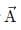then

Detailed Solution for Test: Resolution of Vectors (NCERT) - Question 1

Unit vector is vector with magnitude unity but having specific direction.

Value of unit vector is given by: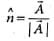Where,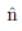= unit vector

A = vector a

∣A∣ = magnitude of vector a

Test: Resolution of Vectors (NCERT) - Question 2

### The components of Vector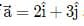​ along the directions of vectors (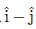) is

Detailed Solution for Test: Resolution of Vectors (NCERT) - Question 2

Given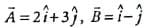, (say) components of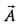along the direction of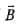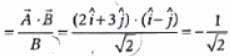Test: Resolution of Vectors (NCERT) - Question 3

### The magnitude of the x-component of vectoris 3 and the magnitude of vectoris 5. What is the magnitude of the y-component of vector?

Detailed Solution for Test: Resolution of Vectors (NCERT) - Question 3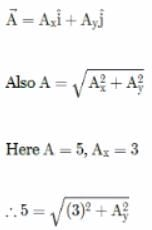Squaring both sides we get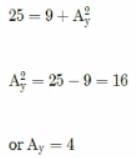Test: Resolution of Vectors (NCERT) - Question 4

The direction cosines of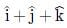are

Detailed Solution for Test: Resolution of Vectors (NCERT) - Question 4

Let∴ Ax = 1, Ay = 1, Az = 1
and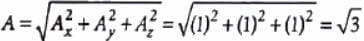cos α, cos β andcos y are the direction cosines of.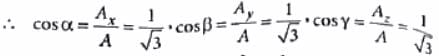Test: Resolution of Vectors (NCERT) - Question 5

If a vectormakes angles α, β and γ  with X, Y and Z axes respectively then sin2α + sin2β + sin2γ is equal to

Detailed Solution for Test: Resolution of Vectors (NCERT) - Question 5

cos2 α + cos2 β + cos2 Y = 1
(1 - sin2 α) + (1 - sin2 β) + (1 - sinY) = 1
or sin2 α + sin2 β + sin2 Y = 3 - 1 = 2

## Physics Class 11

130 videos|483 docs|210 tests
Information about Test: Resolution of Vectors (NCERT) Page
In this test you can find the Exam questions for Test: Resolution of Vectors (NCERT) solved & explained in the simplest way possible. Besides giving Questions and answers for Test: Resolution of Vectors (NCERT), EduRev gives you an ample number of Online tests for practice

## Physics Class 11

130 videos|483 docs|210 tests

### How to Prepare for NEET

Read our guide to prepare for NEET which is created by Toppers & the best Teachers(Scan QR code)